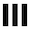##dwitter.net | #fractals

+ New dweet
`function u(t) {`
```} // 122/140 ```

Please log in (or register) to post a dweet (copy-paste the code somewhere safe to save it meanwhile)

``` u(t) is called 60 times per second. t: Elapsed time in seconds. S: Shorthand for Math.sin. C: Shorthand for Math.cos. T: Shorthand for Math.tan. R: Function that generates rgba-strings, usage ex.: R(255, 255, 255, 0.5) c: A 1920x1080 canvas. x: A 2D context for that canvas. ```
Theme challenge of the month: #growth
remix of d/11051 by u/katkip

`function u(t) {`

```}// 138/140 ```

Please log in (or register) to post as a new dweet (copy-paste code somewhere safe to save it meanwhile).

remix of d/4509 by u/ben

`function u(t) {`

```}// 140/140 ```

Please log in (or register) to post as a new dweet (copy-paste code somewhere safe to save it meanwhile).

• blowing in the fractal wind

`function u(t) {`

```}// 133/140 ```

Please log in (or register) to post as a new dweet (copy-paste code somewhere safe to save it meanwhile).

`function u(t) {`

```}// 122/140 ```

Please log in (or register) to post as a new dweet (copy-paste code somewhere safe to save it meanwhile).

• u/iverjo
115 bytes: for(j=1080;j--;a=b=0){for(k=35;k--&&a*a+b*b<4;a=y)y=a*a-b*b+t*60/540-2,b=2*a*b+j/540-1;x.fillRect(t*60,j,1,S(k/9))}
• u/iverjo
Here's one that draws faster: for(i=4;i--;){for(j=1080;j--;a=b=0){for(k=35;k--&&a*a+b*b<4;a=y,l=t*240+i)y=a*a-b*b+l/540-2,b=2*a*b+j/540-1;x.fillRect(l,j,1,S(k/9))}}
• u/iverjo
Nvm, they depend on various variables being defined. I was fooled.
• u/myJS